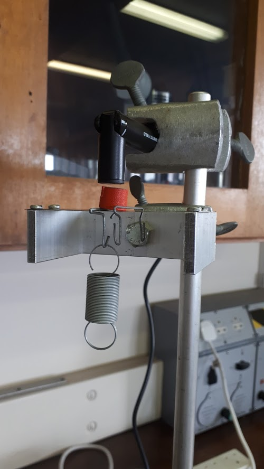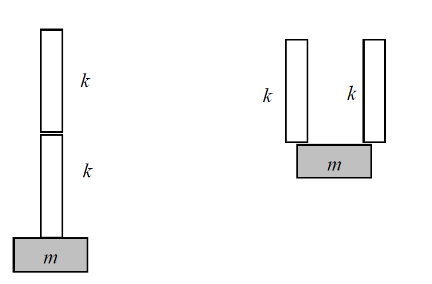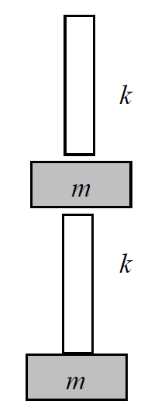# Oscillation Using Springs

A spring exerts a restoring force that is equal in magnitude but opposite in direction to the applied force: F = -k*x where k is the spring constant and has units Nm−1. The term “restoring” force is used because it restores the spring back to its equilibrium (unstrained) position as soon as the applied force is removed.

In an ideal environment (i.e. one without friction or air resistance) the spring would oscillate around the equilibrium position with oscillations of amplitude A. The displacement of a mass on the end of the spring would follow simple harmonic motion.

This experiment builds on the knowledge gained in the two spring experiments in first year but it set-up in a different way. The spring is hung off a metal carrier. A magnet is placed on top of the metal carrier and a magnetic field sensor is placed just above the magnet. When the spring oscillates it pulls the metal carrier up and down which moves the magnet. The movement of the magnet changes the magnetic field, as read by the magnetic field sensor.This experiment is split into three parts:

Part 1: Determine the Spring Constant, k, using Hooke’s Law

Part 1 is to determine the spring constant by means of Hookes Law as done in the first year labs

Part 2: Determine the Spring Constant, k, from the frequency of oscillation

Part 2 is to confirm the spring constant be means of oscillationg the spring which is also done in the first year labs, however in this case the magnet and magnetic field sensor are used to measure the sprind oscillations as opposed to the motion sensor used previously.

Part 3: The damping of a system consisting of one mass and spring

In part 2 we assume that there is no external force on the spring dampening it's motion. However this is not true. There are opposing forces reducing the kinetic energy of the spring and dampening the motion, for example air resistance. As such when looking at the sine wave produced by the oscillations of the spring we can see the amplitude of the wave decreases over time. The dampening of the spring is measured by the reduction in amplitude of the wave.

Part 4: Two springs and one mass: Springs in series and parallel

In this part oscillations in multiple springs undergoing the same force is explored. The images below show the two configurations of springs and weights that are explored to determine the soring constant of the system.Part 5: Two springs and two masses: the motion of two coupled oscillators

When two equal masses and two identical springs are coupled as shown below, the motion appears to be quite complex. In fact, this motion is a simple mixture of two fundamental modes of angularfrequency ω1 and ω2. A fast fourier transform of the oscialltions can pinpoint the frequency of the oscillations. This is carried out for

1. two identical springs and two equal masses
2. two springs and two different masses e.g. 50 g and 100 g.
3. three springs and three identical masses## Department of Physics

Roinn na Fisice

Room 213 (Physics Office), 2nd floor, Kane Science Building, University College Cork, Ireland.,

Top